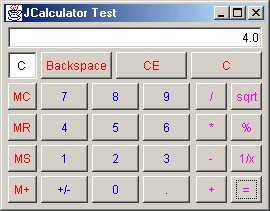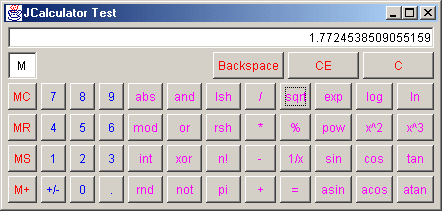##### ORIGINAL DRAFT

Calculators are relatively simple components that can really enhance numerical applications. Tax software, banking applications and even operating systems provide this functionality for the end user, allowing them to do quick calculations from a pop up, without interrupting program flow. JCalculator can provide both simple and advanced calculator functionality for your Swing applications.Figure 1: Standard JCalculator display.

JCalculator is a stack-based calculator, not unlike the accessories provided in typical operating systems. Our implementation provides both a compact mode, as seen in Figure 1, and an extended mode, as seen in Figure 2. In both cases, a standard memory storage and recall feature is provided, along with basic numerical entry and functions. Both unary and binary functions are supported, over thirty in total.Figure 2: Expanded JCalculator display.

If you take a look at Figure 3, you can get a sense of the architecture of this design. I’ve put a number of classes and interfaces inside the CalculatorCommands class, because they were developed as inner classes. We use the Command pattern and extend a number of additional interfaces to take care of the functions, both unary and binary. Some of the functionality directly implements the Command interface, such as the memory capabilities, but most of the operations are part of the Function subset of classes.

In many ways the subclassed interfaces exist to distinguish between functional behaviors which can be branched on. For example, if we know we are dealing with a Unary function, we can push the operand on the stack, followed by the operator. In the case of a binary function, the stack will have two operands. In the case of a basic command, no operands are expected.Figure 3: JCalculator classes.

There are a large number of operations implemented as commands, unary or binary functions. Each is an inner class of CalculatorCommands. To make these easy to use, the CalculatorButton class allows you to pass a Command as an argument and takes care of calling appropriate functionality. It is, in fact, the CalculatorCommands classes which decide what to do with the stack and each operation.

JCalculator make use of a single CalculatorStack object and a pair of CalculatorField instances - one for the display field and the other a state marker for the memory commands. If the memory is set, this field will display the letter ‘M’, otherwise ‘C’ will be displayed to imply that memory is clear. The rest of the JCalculator display is made up of buttons.

Listing 1 shows the CalculatorButton class. Other than the standard button text, we pass a reference to JCalculator and a Command instance in the constructor. CalculatorButton implements it’s own action listener and responds directly to button clicks, so we register as an ActionListener. We also set small margins and disable the ability to become the default button.

You’ll notice that we override the paintComponent method and call the superclass implementation after setting the foreground color. This provides an easy way to set text color in button groups rather than having to set them individually. By approaching the problem this way, panels that group function, memory, or other logical combinations can be set to colors that distinguish them from other button groups without requiring lots of repeated code. While these aren’t intended as primary visual cues, they can make it easier for the end-user to recognize logical separation and grouping.

The actionPerformed method does all the real work in the CalculatorButton class. We ignore conditions in which the command is set to null, and use conditional statements to distinguish between Unary, Binary and basic Command operations. In the case of a null command, we call the evaluate method to make sure the stack is effectively processed. By default, the equal button simply uses a null command. Standard commands like the memory support call the exec method from the Command interface directly.

When we’re dealing with a Unary function, we simply get a handle to the CalculatorStack and push a single operand onto it, calling evaluate to do the actual calculation. For Binary functions, we push the currently displayed number onto the stack, and then the function, as we do in the case of a Unary function, and then we clear the field.

The evaluate method is fairly simple. It gets the current field value, pops the function off the stack and pushes the current field value onto the stack. If the function was Unary, the field value is the only value on the stack. If it was Binary, there are two values, the previous and current field values. The Command’s exec method is then called and the result is placed on the stack, replacing any previous arguments. We can then pop the result off the stack and put it in the display field to show the output.

Listing 2 shows part of the code for CalculatorCommands. I’ve taken the liberty of removing most of the Command and Function implementations for publication, because they are fundamentally so similar. You’ll find the complete code online at www.java-pro.com, with all the functions intact. In effect, we declare a number of interfaces, which extend the Command interface. This allows us to use the instanceof operator to distinguish between the classes.

Listed first are the Binary functions. I’ve left the Plus class there for illustration. All Binary functions follow the same basic pattern. They pop two numbers off the stack and operate on them. The only thing worthy of notice is the order in which the operands are handled. The first number popped off the stack is the second number to be operated on. This is not an issue in Unary functions, since only one operand is required. I’ve left the Percent class for illustration purposes. The final inner class is Clear, which implements the Command interface directly and merely get a reference to the display field and clears it.

JCalculator, shown in Listing 3, ties everything together in a JPanel, so you can include it in any Swing container. Other than setting up a couple of CalculatorField instances and the CalculatorStack, most of the work is in esthetic button placement. There are a set of convenience methods to set up button panels for support buttons, basic math functions, each of the digit buttons, general functions, trigonometry and the memory commands. Each of these relies on the CalculatorCommands inner classes for actual execution. The rest of the class merely implements accessor methods so that developers and Command implementations can easily access JCalculator properties.

JCalculator provides your with another component for your user interface bag of tricks. It’s not likely to be critical to any application but it has the ability to enhance the right kind of program in meaningful ways. If your application makes heavy use of numbers, this may be just what you’re looking for to facilitate the user experience.

Listing 1

``````import java.awt.*;
import java.awt.event.*;
import javax.swing.*;

public class CalculatorButton extends JButton
implements ActionListener
{
protected CalculatorCommands.Command command;

protected JCalculator calculator;

public CalculatorButton(
String text, JCalculator calculator,
CalculatorCommands.Command command)
{
super(text);
this.calculator = calculator;
this.command = command;
setMargin(new Insets(2, 2, 2, 2));
setDefaultCapable(false);
}

public void paintComponent(Graphics g)
{
setForeground(getParent().getForeground());
super.paintComponent(g);
}

public void actionPerformed(ActionEvent event)
{
if (command != null)
{
if (command instanceof CalculatorCommands.Unary)
{
evaluate();
CalculatorStack stack = calculator.getStack();
stack.pushFunction((CalculatorCommands.Function)command);
evaluate();
}
if (command instanceof CalculatorCommands.Binary)
{
evaluate();
CalculatorField field = calculator.getField();
CalculatorStack stack = calculator.getStack();
stack.pushNumber(field.getNumber());
stack.pushFunction((CalculatorCommands.Function)command);
field.clearField();
}
if (!(command instanceof CalculatorCommands.Function))
{
command.exec(calculator);
}
}
// Handle '='
else evaluate();

}

protected void evaluate()
{
CalculatorStack stack = calculator.getStack();
if (!stack.isEmpty() && stack.isFunction())
{
CalculatorField field = calculator.getField();
CalculatorCommands.Function function = stack.popFunction();
stack.pushNumber(field.getNumber());
function.exec(calculator);
field.setNumber(stack.popNumber());
}
}
}
``````

Listing 2

``````import java.awt.*;
import javax.swing.*;

public class CalculatorCommands
{
public static interface Command
{
public void exec(JCalculator calculator);
}

public static interface Function extends Command {}

public static interface Unary extends Function {}

public static interface Binary extends Function {}

// ----------------------------------------------------------------
// BINARY FUNCTIONS
// ----------------------------------------------------------------

public static class Plus implements Binary
{
public void exec(JCalculator calculator)
{
CalculatorStack stack = calculator.getStack();
double field = stack.popNumber();
stack.pushNumber(stack.popNumber() + field);
}
}

// CODE REMOVED FOR PRINT... SEE ONLINE SOURCE CODE...

// ----------------------------------------------------------------
// UNARY FUNCTIONS
// ----------------------------------------------------------------

public static class Percent implements Unary
{
public void exec(JCalculator calculator)
{
CalculatorStack stack = calculator.getStack();
stack.pushNumber(stack.popNumber() / 100);
}
}

// CODE REMOVED FOR PRINT... SEE ONLINE SOURCE CODE...

// ----------------------------------------------------------------
// COMMANDS
// ----------------------------------------------------------------

public static class Clear implements Command
{
public void exec(JCalculator calculator)
{
CalculatorField field = calculator.getField();
field.clearField();
}
}

// CODE REMOVED FOR PRINT... SEE ONLINE SOURCE CODE...

}
``````

Listing 3

``````import java.awt.*;
import java.util.*;
import javax.swing.*;

public class JCalculator extends JPanel
implements SwingConstants
{
protected CalculatorField field = new CalculatorField();
protected CalculatorStack stack = new CalculatorStack();
protected CalculatorField label = new CalculatorField("C");
protected JPanel math, trig;
protected boolean expand;
protected double memory;

public JCalculator()
{
this(false);
}

public JCalculator(boolean expand)
{
label.setHorizontalAlignment(CENTER);

JPanel exp = new JPanel(new BorderLayout(4, 4));
setExpand(expand);

JPanel main = new JPanel(new BorderLayout(4, 4));

setBorder(BorderFactory.createEmptyBorder(4, 4, 4, 4));
setLayout(new BorderLayout(4, 4));
}

protected JPanel createClearPanel()
{
JPanel panel = new JPanel(new GridLayout(1, 4, 4, 4));
panel.setForeground(Color.red);
this, new CalculatorCommands.Backspace()));
this, new CalculatorCommands.Clear()));
this, new CalculatorCommands.ClearAll()));
JPanel east = new JPanel(new BorderLayout());
return east;
}

protected JPanel createDigitPanel()
{
JPanel panel = new JPanel(new GridLayout(4, 3, 4, 4));
panel.setForeground(Color.blue);
this, new CalculatorCommands.Seven()));
this, new CalculatorCommands.Eight()));
this, new CalculatorCommands.Nine()));
this, new CalculatorCommands.Four()));
this, new CalculatorCommands.Five()));
this, new CalculatorCommands.Six()));
this, new CalculatorCommands.One()));
this, new CalculatorCommands.Two()));
this, new CalculatorCommands.Three()));
this, new CalculatorCommands.Sign()));
this, new CalculatorCommands.Zero()));
this, new CalculatorCommands.Decimal()));
return panel;
}

protected JPanel createFunctionPanel()
{
JPanel panel = new JPanel(new GridLayout(4, 2, 4, 4));
panel.setForeground(Color.magenta);
this, new CalculatorCommands.Div()));
this, new CalculatorCommands.Sqrt()));
this, new CalculatorCommands.Mult()));
this, new CalculatorCommands.Percent()));
this, new CalculatorCommands.Minus()));
this, new CalculatorCommands.Reciprocal()));
this, new CalculatorCommands.Plus()));
return panel;
}

protected JPanel createMathPanel()
{
JPanel panel = new JPanel(new GridLayout(4, 3, 4, 4));
panel.setForeground(Color.magenta);
this, new CalculatorCommands.Abs()));
this, new CalculatorCommands.And()));
this, new CalculatorCommands.Left()));

this, new CalculatorCommands.Mod()));
this, new CalculatorCommands.Or()));
this, new CalculatorCommands.Right()));

this, new CalculatorCommands.Int()));
this, new CalculatorCommands.Xor()));
this, new CalculatorCommands.Factorial()));

this, new CalculatorCommands.Round()));
this, new CalculatorCommands.Not()));
this, new CalculatorCommands.PI()));

return panel;
}

protected JPanel createTrigPanel()
{
JPanel panel = new JPanel(new GridLayout(4, 3, 4, 4));
panel.setForeground(Color.magenta);
this, new CalculatorCommands.Exp()));
this, new CalculatorCommands.Log()));
this, new CalculatorCommands.Ln()));

this, new CalculatorCommands.Pow()));
this, new CalculatorCommands.Square()));
this, new CalculatorCommands.Cube()));

this, new CalculatorCommands.Sin()));
this, new CalculatorCommands.Cos()));
this, new CalculatorCommands.Tan()));

this, new CalculatorCommands.Asin()));
this, new CalculatorCommands.Acos()));
this, new CalculatorCommands.Atan()));
return panel;
}

protected JPanel createMemoryPanel()
{
JPanel panel = new JPanel(new GridLayout(5, 1, 4, 4));
panel.setForeground(Color.red);
this, new CalculatorCommands.MemClear()));
this, new CalculatorCommands.MemRecall()));
this, new CalculatorCommands.MemStore()));
this, new CalculatorCommands.MemPlus()));
return panel;
}

public CalculatorField getField()
{
return field;
}

public CalculatorStack getStack()
{
return stack;
}

public CalculatorField getLabel()
{
return label;
}

public double getMemory()
{
return memory;
}

public void setMemory(double memory)
{
this.memory = memory;
}

public boolean isExpand()
{
return expand;
}

public void setExpand(boolean expand)
{
this.expand = expand;
math.setVisible(expand);
trig.setVisible(expand);
invalidate();
}
}
``````